# Uniform stability

(diff) ← Older revision | Latest revision (diff) | Newer revision → (diff)

Lyapunov stability, uniform with respect to the initial time. A solution,, of a system of differential equationsis called uniformly stable if for everythere is a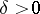such that for every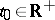and every solution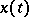of the system satisfying the inequality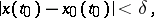the inequality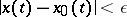holds for all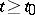.

A Lyapunov-stable fixed point of an autonomous system of differential equations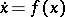,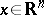, is uniformly stable, but, in general, a Lyapunov-stable solution need not be uniformly stable. For example, the solution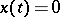,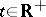, of the equation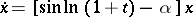(1)

is stable for each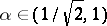but is not uniformly stable for such.

Suppose one is given a linear system of differential equations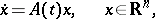(2)

where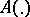is a mapping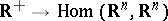that is summable on each interval.

In order that the solution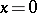of (2) be uniformly stable, it is necessary that the upper singular exponent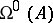of (2) be less than or equal to zero (cf. also Singular exponents). For example, in the case of equation (1), the upper singular exponent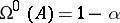, and the Lyapunov characteristic exponent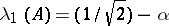. For the existence of asuch that the solutionof any system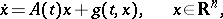that satisfies the conditions of the existence and uniqueness theorem for the solution of the Cauchy problem as well as the condition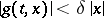be uniformly stable, it is necessary and sufficient that the upper singular exponentbe less than zero.

How to Cite This Entry:
Uniform stability. Encyclopedia of Mathematics. URL: http://encyclopediaofmath.org/index.php?title=Uniform_stability&oldid=17527
This article was adapted from an original article by V.M. Millionshchikov (originator), which appeared in Encyclopedia of Mathematics - ISBN 1402006098. See original article